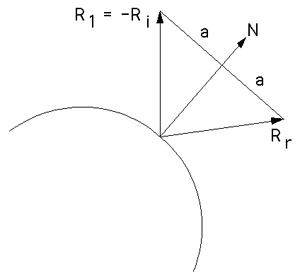# Calculating reflected ray

Written by Paul Bourke
January 2000

This short note gives the equation for a reflected ray as used in many computer rendering applications, eg: ray tracing. Given a ray Ri incident at a point on a surface with normal N one wishes to determine the reflected ray from that point. The geometry and nomenclature is given in the following diagram.The result is determined by straightforward geometry as follows where "." indicates the dot product and typically N and R1 are unit vectors.

 Rr = N (R1 . N) + a and R1 + a = N (R1 . N) so Rr = 2 N (R1 . N) - R1
If -Ri is substituted for R1 then we have
 Rr = Ri - 2 N (Ri . N)

Some obvious things to note at extreme cases. If Ri is parallel to the normal then Rr = N, that is, the reflected ray is in the opposite direction to the incident ray. If the incident ray is perpendicular to the normal then it is unaffected, Rr = Ri, this is a glancing ray.

Note also that while the above diagram is in 2D, the equations hold for any dimension.